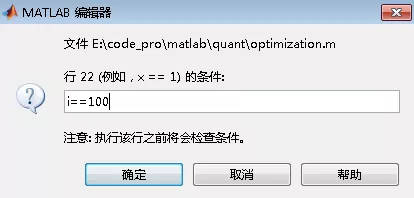#Rex engineer知乎上有个“有哪些让人相见恨晚的Matlab命令”的话题，很多答主提供的命令确实很实用，为了更方便大家的学习，我就知乎上的答案和我自己想到的都综合整理成了一篇文章，把我觉得很实用的指令整理出来。

### dbstop if error

如果运行出错，matlab会自动停在出错的那一行，并保存相关变量，非常好用的指令，谁用谁知道。

### linkaxes

当使用`subplot`作图时，想要同时缩放多幅图，就可以使用该指令

``````figure;
ax(1) = subplot(211);
plot(sig1);ylim([-2,2]);grid on
ax(2) = subplot(212);
plot(sig2);ylim([-2,2]);grid on
linkaxes(ax, 'xy');                    %x轴和y轴都同步，也可以单独指定x或者y
``````

### yyaxis right

当我们要把两个波形画到一个图中时，大家都知道可以使用`hold on`，但如果两个波形的值域相差很大时，如下：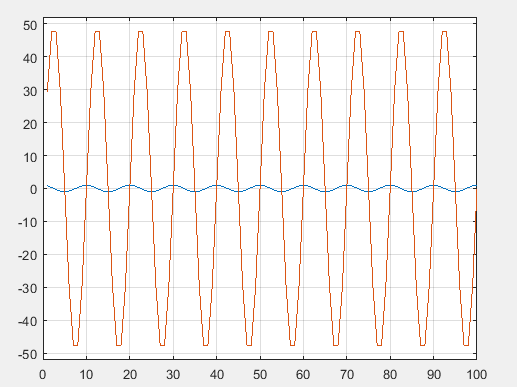如果我们使用了`yyaxis right`

``````plot(sig1);
yyaxis right
plot(sig2);
``````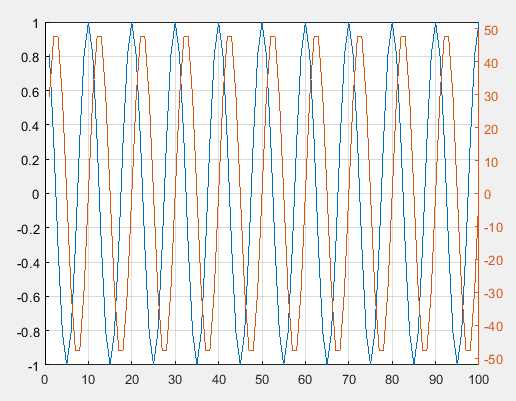### 折叠注释内容

使用下面的注释方式可以将注释后的内容折叠起来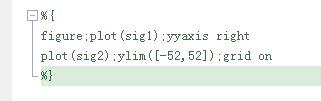### deal

可简化初始化的代码

``````% 要初始化4个矩阵
A = zeros(3,4);
B = zeros(3,4);
C = zeros(3,4);
D = zeros(3,4);
``````

``````[A,B,C,D] = deal(zeros(3,4));
``````

``````%% 原始程序
A = zeros(3,4);
B = [];
C = ones(2);
D = cell(2);
%% 使用deal的程序
[A,B,C,D] = deal(zeros(3,4), [], ones(2), cell(2));
``````

### 查看Matlab函数源代码

有时觉得自己写的程序不规范，想参考Matlab官方是怎么写的，比如想看SVM分类器是怎么写的，可以输入

``````edit ClassificationSVM.m
``````

### fdatool

设计滤波器必备利器，在Matlab2019中还能用，但提示以后要换成`filterDesigner`了，设计完滤波器后可以把系数导出，还能直接导出成Xilinx FPGA开发软件可以识别的coe文件。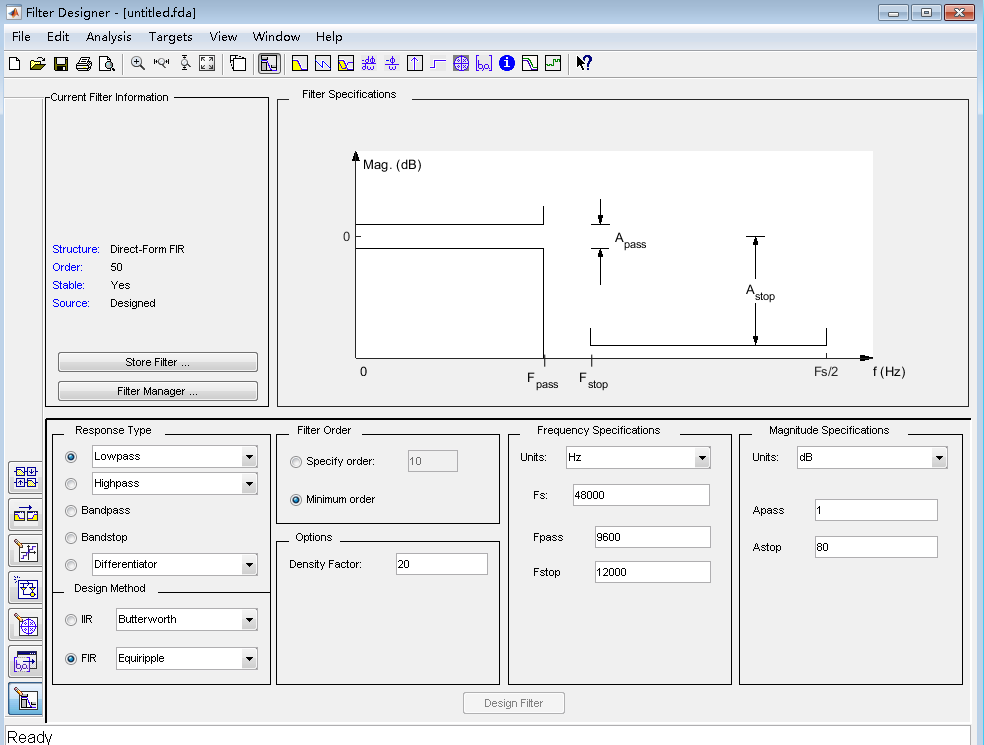### 条件断点

``````n = 1000;
for i=1:n
xxx
end
``````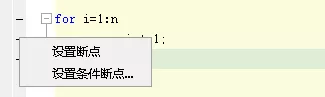再设置我们想要的条件就好了